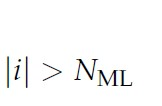# Greater than or equal to condition "modulus" in matlab

how to writegreater than or equal to condition and “modulus” in matlab inside CVXIf `i` is a CVX variable or expression, that constraint is non-convex.

However, you may be able to model it (actually with `>=`, not `>`) in CVX by using binary variables, using CVX’s MIDCP capability. If `i` ;is real, that’s just `abs(i)`. So use the Big M model for `abs` in https://yalmip.github.io/tutorial/logicprogramming, which you need to use because you are using `abs` in a non-convex way (inequality is going the wrong direction to be convex).

If `i`is complex, you might be out of luck using CVX or any convex optimization tool. But if someone knows how to do it, please post. Well, maybe there’s a messy way of linearizing it per component.

1 Like

thank you Dr Mark_L_Stone for helping us in CVX. We appreciate your effort with us